# Quasi-3D solar cell solver¶

﻿The quasi-3D solar cell model included in Solcore uses a SPICE-based electrical network to model the flow of injected current through the solar cell. The plane of the cell is discretized into many elements, each of them representing a small portion of the cell. Depending on the location of the element - exposed to the sunlight or underneath a metal finger - the IV curve of the cell will be the light IV or the dark IV. Each element is linked to their neighbours with resistors, representing the lateral current flow and dependent on the sheet resistance of the cells. This method can be applied to any number of junctions.

This type of formalism is widely used to simulate the performance of solar cells when the effect of a spatial variable needs to be incorporated in the model. This variable can be the design of the front metal grid, in order to minimise the effect of series resistances; the inhomogeneous illumination profile in concentrator devices; the impact of such inhomogeneity on the transport through the tunnel junctions; or the distribution of defects or inhomogeneities. Recently, this formalism was used to model the photoluminescence and the electroluminescence based IV curves of MJ devices, accounting for the limited lateral carrier transport.

Specifically for the modelling and optimization of the front grid of solar cells in order to minimise shading losses and series resistance, there are two packages already available: PVMOS, developed by B. E. Pieters in C and released as open source, and Griddler, developed by J. Wong using Matlab and available at PV Lighthouse.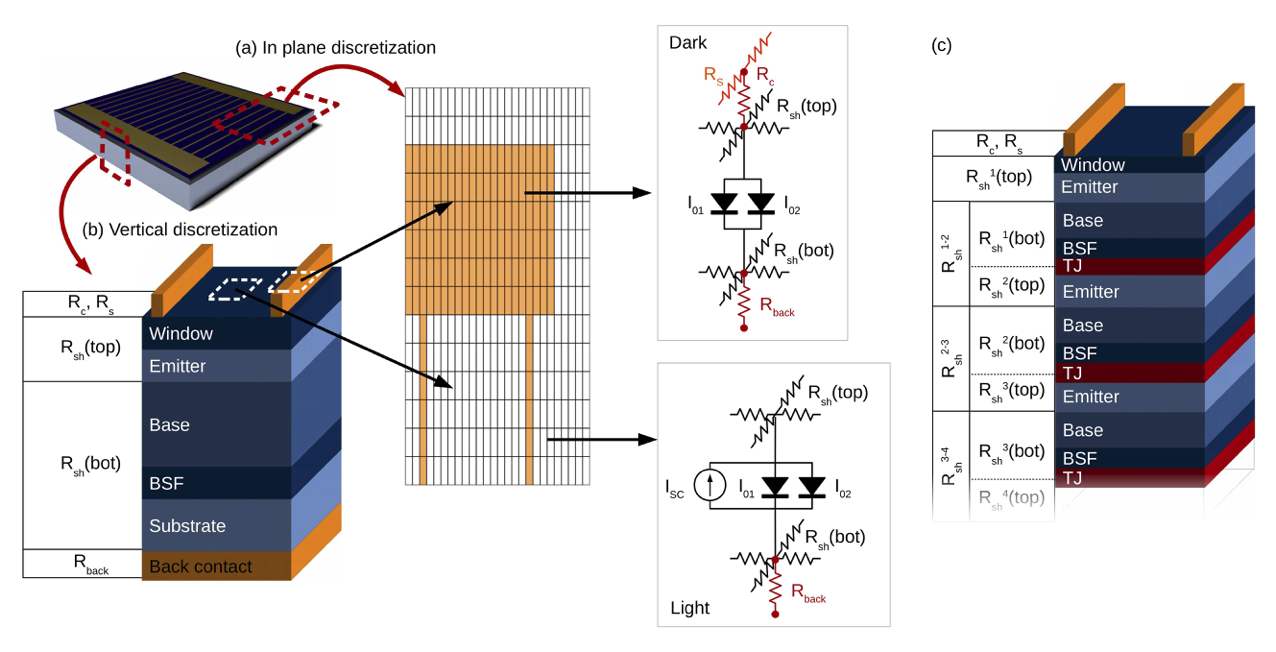## In-plane discretization¶

There are two regions in the plane: the metal and the aperture. These two are provided to Solcore as grey scale images that will work as masks. The resolution of the images, in pixels, will define the in-plane discretization. By default, the aspect ratio of the pixels in the image will be 1:1, but this can be set to a different value in order to reduce the number of elements and improve speed. For example, the in-plane discretization of Fig. [fig:spice_overview]a has an aspect ratio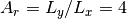, with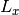and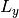the pixel size in each direction.

The values of the pixels in the metal mask are <55 where there is no metal (the aperture area), >200 where there is metal and the external electrical contacts (the boundaries with fixed, externally set voltage values) and any other value in between to represent regions with metal but not fixed voltage. The pixels of the illumination mask - which become the aperture mask after removing the areas shadowed by the metal - can have any value between 0 and 255. These values divided by 255 will indicate the intensity of the sunlight at that pixel relative to the maximum intensity.

The minimum total number of nodes where SPICE will need to calculate the voltages will be N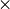M2Q, with N and M the number of pixels in both in-plane directions and Q the number of junctions, which require 2 nodes each. To this, the front and back metal contacts could add a maximum of 2(NM) nodes. Exploiting symmetries of the problem as well as choosing an appropriate pixel aspect ratio will significantly reduce the number of nodes and therefore the time required for the computation of the problem.

## Vertical discretization¶

First, the solar cell is solved in order to obtain the parameters for the 2-diode model at the given illumination conditions. These parameters are then used to replicate the 2-diode model in SPICE. The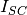is scaled in each pixel by the intensity of the illumination given by the illumination mask. Sheet resistances above and below each junction,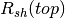and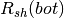, account for the lateral transport. Beneath the metal, there is no current source, as the region is in the dark, and there are extra resistances accounting for the contact between the metal and the semiconductor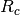and the transport along the metal finger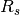. Given that the pixels can be asymmetric, these resistances need to be defined in both in-plane directions,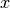and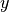: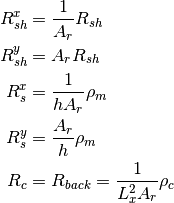where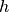is the height of the metal,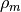their linear resistivity and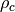the contact resistivity between metal and semiconductor. The sheet resistance of a stack of semiconductor layers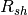is equal to the combination in parallel of the individual sheet resistances. Using the single junction example of the figure,will be given by: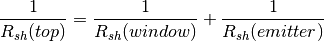Each of these can be estimated from the thickness of the layer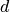, the majority carrier mobility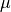and the doping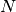as: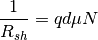If the solar cell has been defined using only the DA and PDD junction models, this information is already available for all the layers of the structure. For junctions using the DB and two diode models,will need to be provided for the top and bottom regions of each junction. Intrinsic layers will be ignored as they do not contribute to the lateral current transport.

## Quasi-3D solver functions¶

solcore.spice.quasi_3D_solver.solve_quasi_3D(solar_cell, injection, contacts, options=None, Lx=1e-05, Ly=1e-05, h=2e-06, R_back=1e-16, R_contact=1e-16, R_line=1e-16, bias_start=0, bias_end=1.8, bias_step=0.01)[source]

Entry function for the quasi-3D solver

Parameters: solar_cell – A solar cell object injection – 2D array indicating the (optical) injection mask contacts – 2D array indicating the electrical contacts options – Options for the 1D solar cell solver Lx – Pixel size in the X direction Ly – Pixel size in the Y direction h – Height of the metal fingers R_back – Resistance back contact R_contact – Contact resistance R_line – Resistivity metal fingers bias_start – Initial voltage (V) bias_end – Final voltage (V) bias_step – Voltage step (V) A tuple with: V [steps + 1] : 1D Array with the external voltages I [steps + 1] : 1D Array with the current at all external V Vall [xnodes, ynodes, 2 * junctions, steps + 1] : 4D Array with the voltages in all nodes, at all external V Vmet [xnodes, ynodes, steps + 1] : 3D Array with the voltages in the metal nodes, at all external V
solcore.spice.quasi_3D_solver.create_node(type, idx, idy, Lx, Ly, Isc, topLCL, botLCL, rshunt, rseries, xMetalTop, yMetalTop, contact)[source]

Creates a node of the solar cell, meaning all the circuit elements at an XY location in the plane. This includes all the diodes, resistances and current sources for all the junctions at that location.

Parameters: type – The type of the node, ‘Normal’, ‘Finger’ or ‘Bus’ idx – Index with the location in the X direction idy – Index with the location in the Y direction Lx – Pixel size in the X direction Ly – Pixel size in the Y direction Isc – Array of Isc for each of the junctions topLCL – Array of resistances of the top lateral conductive layer botLCL – Array of resistances of the bottom lateral conductive layers rshunt – Array of Rshunt for each of the junctions rseries – Array of Rseries for each of the junctions xMetalTop – Resistance of the metal in the X direction yMetalTop – Resistance of the metal in the Y direction contact – Contact resistance The node define in SPICE file as a string.
solcore.spice.quasi_3D_solver.create_header(I01, I02, n1, n2, Eg, T=20)[source]

Creates the header of the SPICE file, where the diode models, the temperature and the independent voltage source are defined.

Parameters: I01 – Array of I01 for each of the junctions I02 – Array of I02 for each of the junctions n1 – Array of n1 for each of the junctions n2 – Array of n2 for each of the junctions Eg – Array of Eg for each of the junctions T – Temperature of the device The header of the SPICE file as a string.
solcore.spice.quasi_3D_solver.solve_circuit_quasi3D(vini, vfin, step, Isc, I01, I02, n1, n2, Eg, Rshunt, Rseries, injection, contacts, RsTop, RsBot, Rline, Rcontact, Lx, Ly)[source]

This is the function that actually dumps all the information to the Spice engine, runs the calculation, and retrieves the datafrom the calculator.

Parameters: vini – Initial voltage (V) vfin – Final voltage (V) step – Voltage step (V) Isc – Array of Isc for each of the junctions I01 – Array of I01 for each of the junctions I02 – Array of I02 for each of the junctions n1 – Array of n1 for each of the junctions n2 – Array of n2 for each of the junctions Eg – Array of Eg for each of the junctions Rshunt – Array of Rshunt for each of the junctions Rseries – Array of Rseries for each of the junctions injection – 2D array indicating the (optical) injection mask contacts – 2D array indicating the electrical contacts RsTop – Array of sheet resistance on the top for each of the junctions RsBot – Array of sheet resistance on the bottom for each of the junctions Rline – Resistance of the metal fingers Rcontact – Contact resistance Lx – Pixel size in the X direction Ly – Pixel size in the Y direction A tuple with: V [steps + 1] : 1D Array with the external voltages I [steps + 1] : 1D Array with the current at all external V Vall [xnodes, ynodes, 2 * junctions, steps + 1] : 4D Array with the voltages in all nodes, at all external V Vmet [xnodes, ynodes, steps + 1] : 3D Array with the voltages in the metal nodes, at all external V